# RD Sharma Solutions for Class 12 Maths Chapter 10 Differentiability

## RD Sharma Solutions for Class 12 Maths Chapter 10 – Free PDF Download Updated for (2021-22)

RD Sharma Solutions for Class 12 Maths Chapter 10 – Differentiability is provided here for the benefit of the students. To achieve a good academic score in Mathematics, the important thing to be done by the students is to practice all the questions of each and every exercise. Students can score good marks in the exams by solving and cross-checking the answers with the RD Sharma Solutions prepared by BYJU’S experts in Maths.

RD Sharma Solutions for Class 12 Maths are focused on learning various mathematical tricks and shortcuts for quick and easy calculations. These solutions are in accordance with the latest CBSE syllabus and marking schemes. Students can easily access the PDF of RD Sharma Solutions for Class 12 Maths Chapter 10 Differentiability from the given links.

Let us have a look at some of the important concepts that are discussed in the RD Sharma Solutions of this chapter.

• Differentiability at a point
• Definition and meaning of differentiability at a point
• Differentiability in a set
• Some useful results on differentiability

## RD Sharma Solutions For Class 12 Maths Chapter 10 Differentiability:-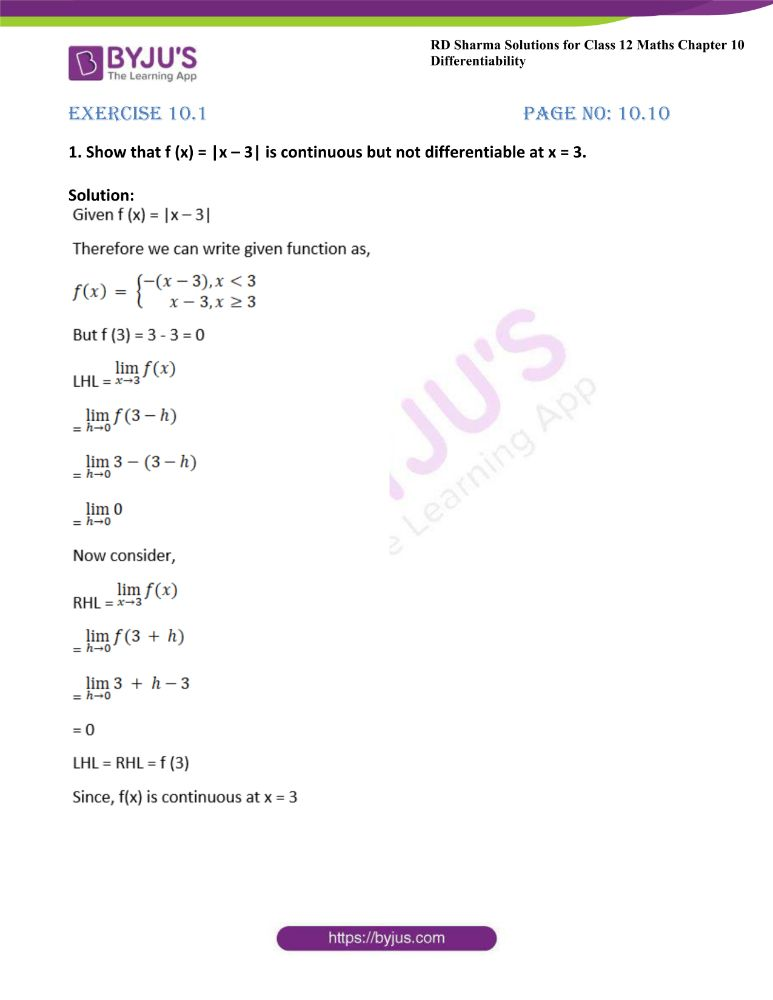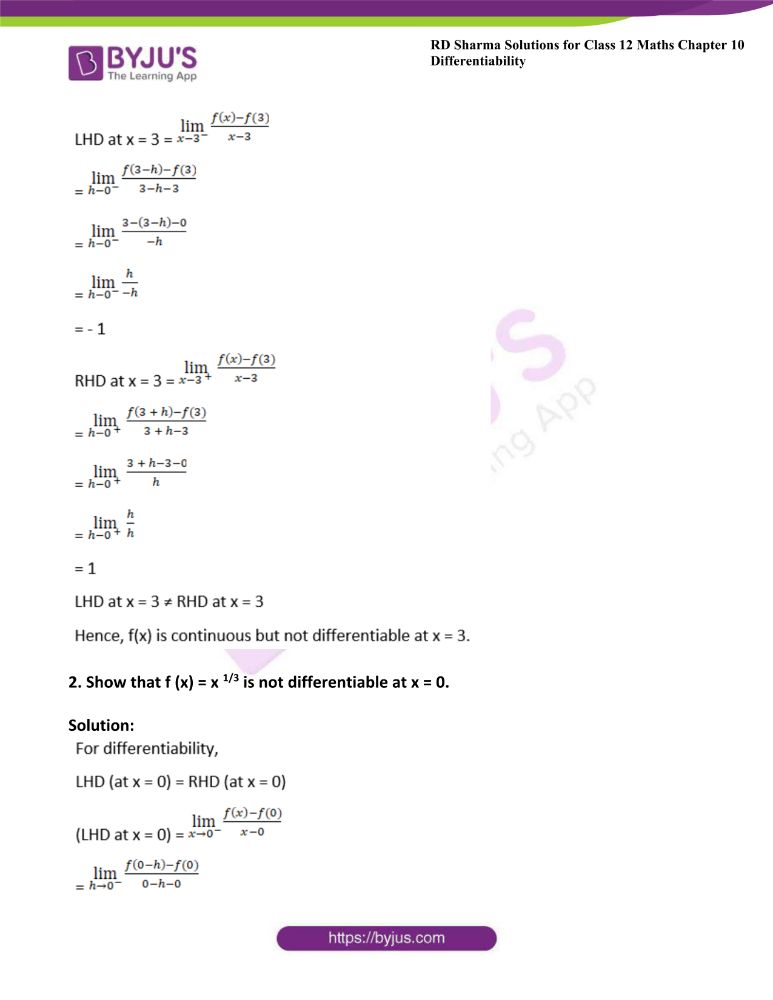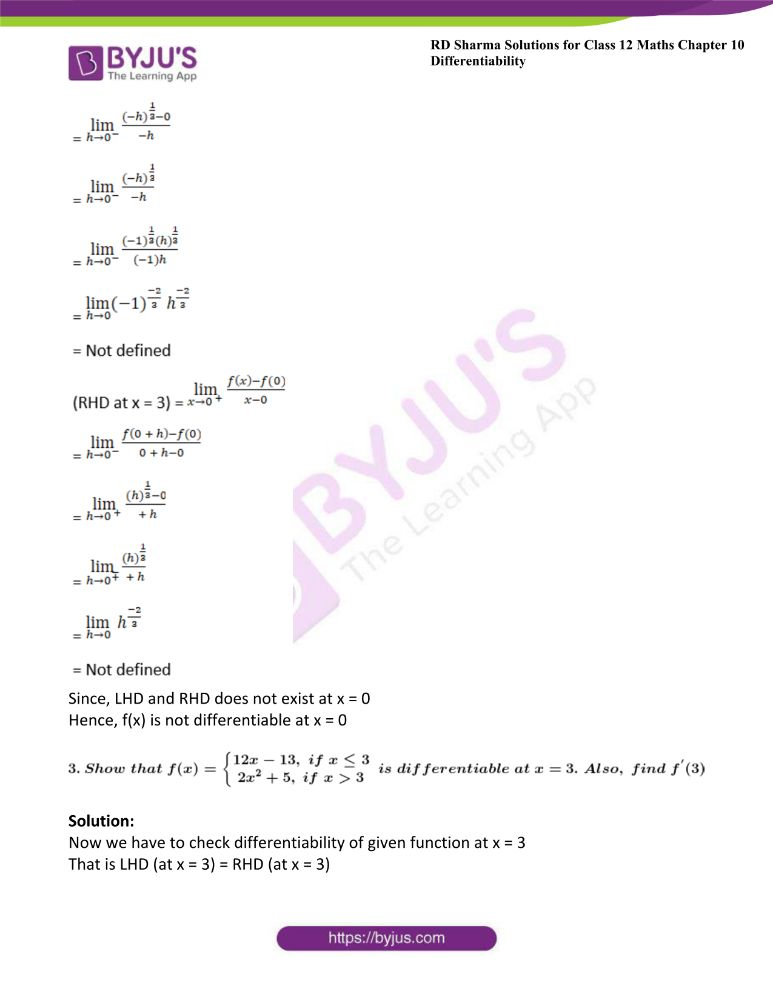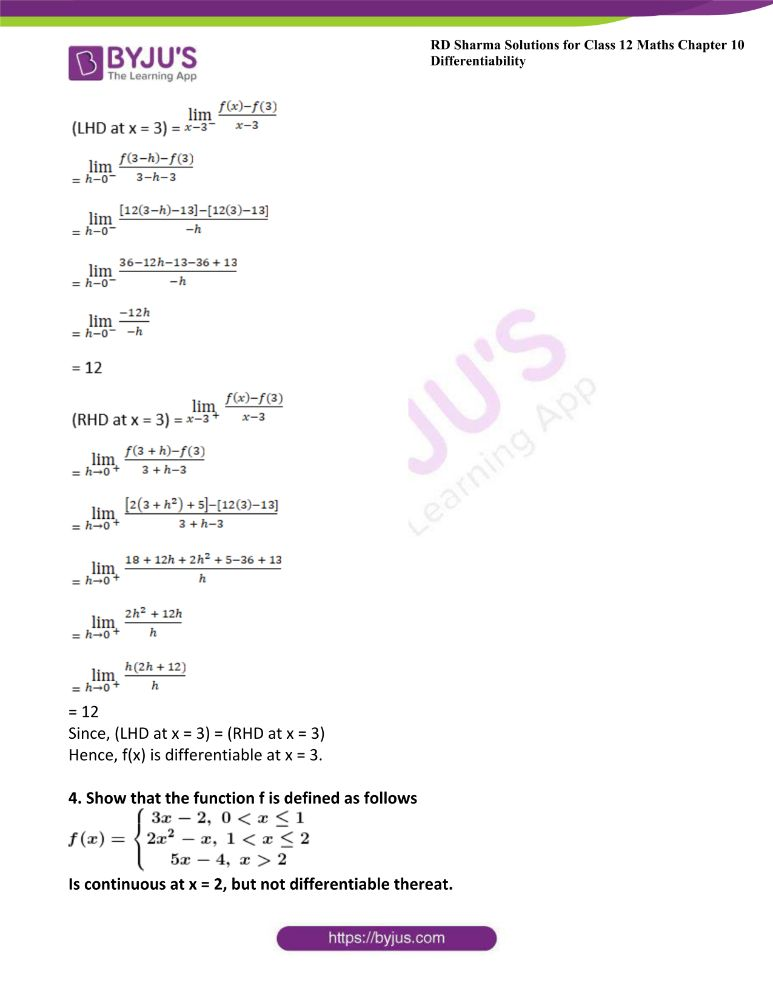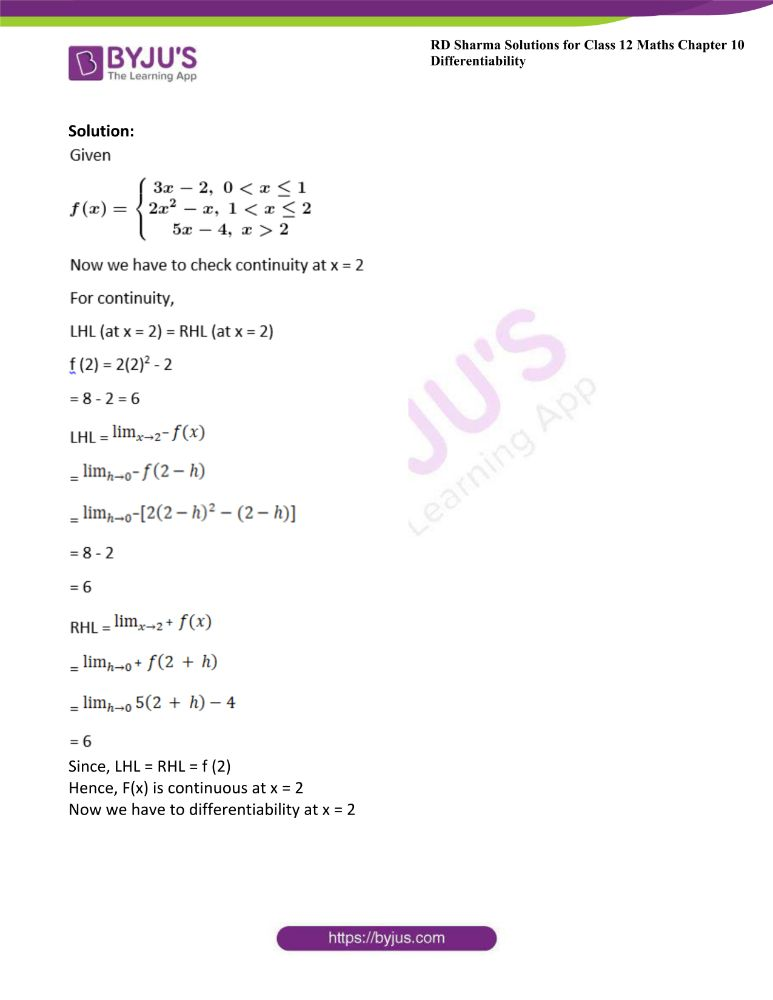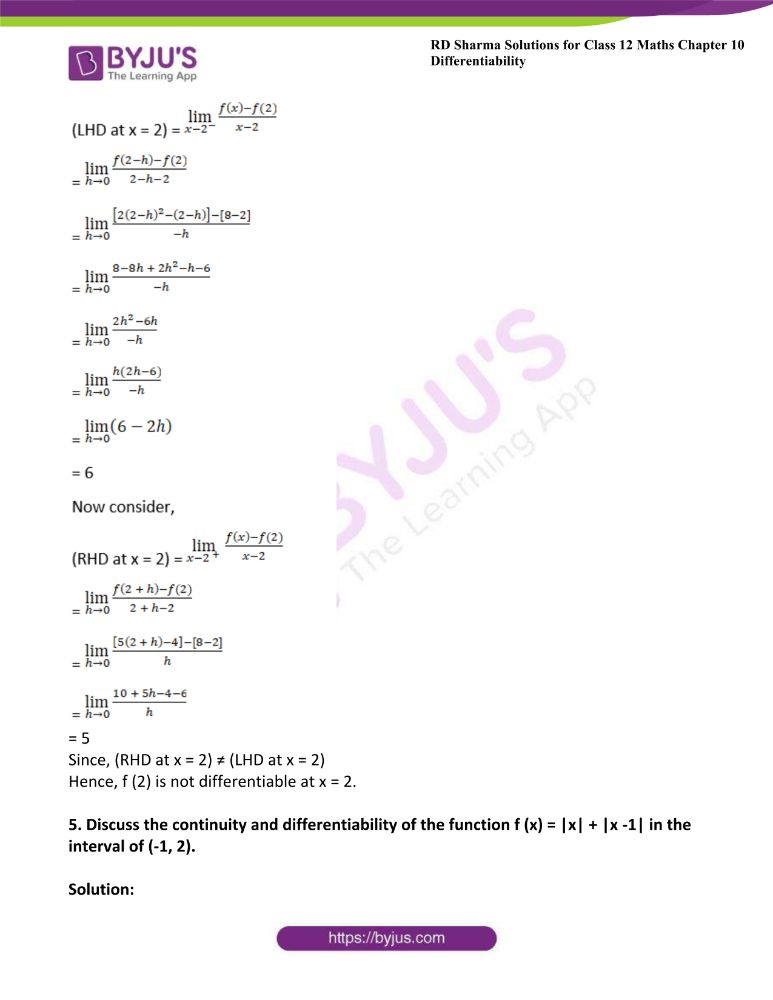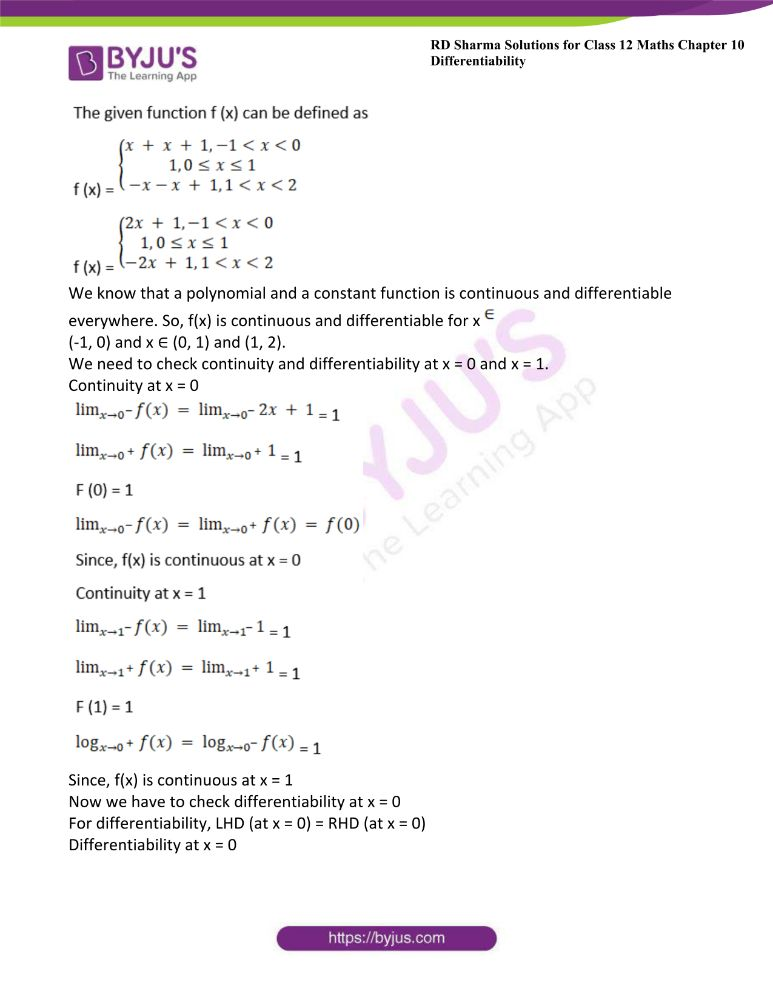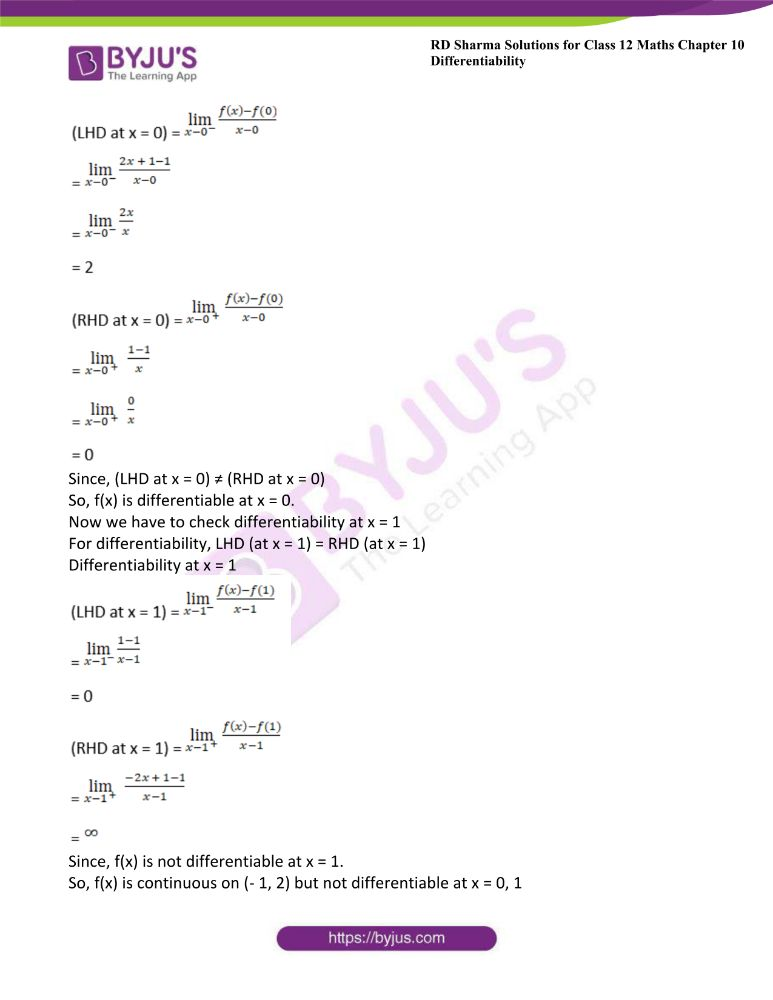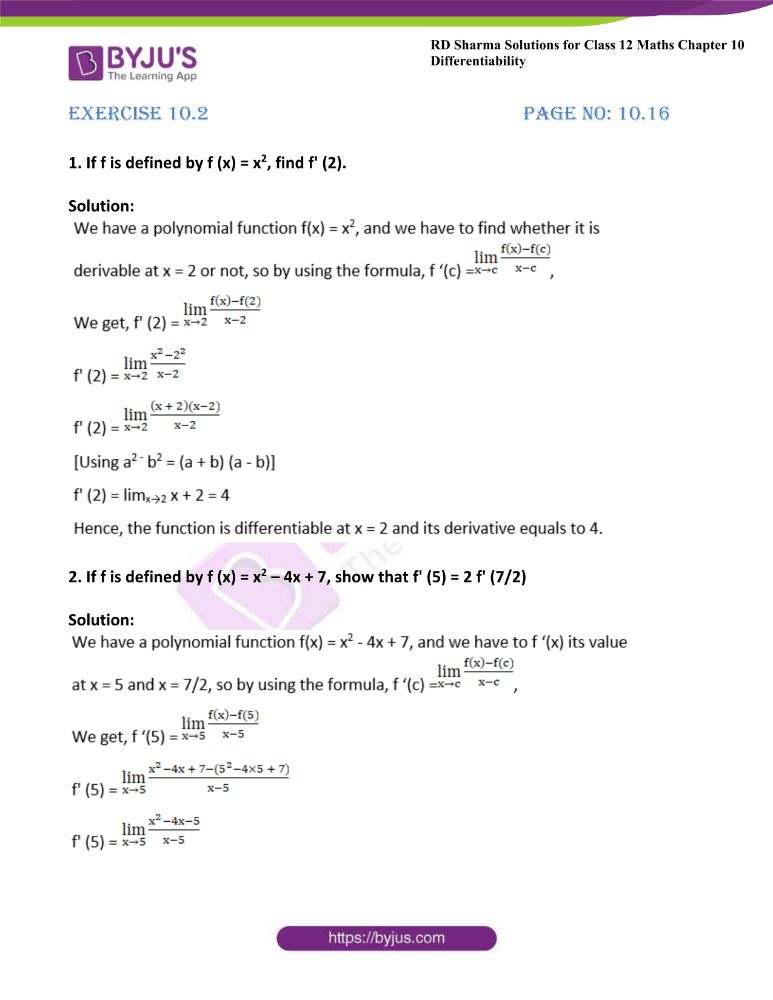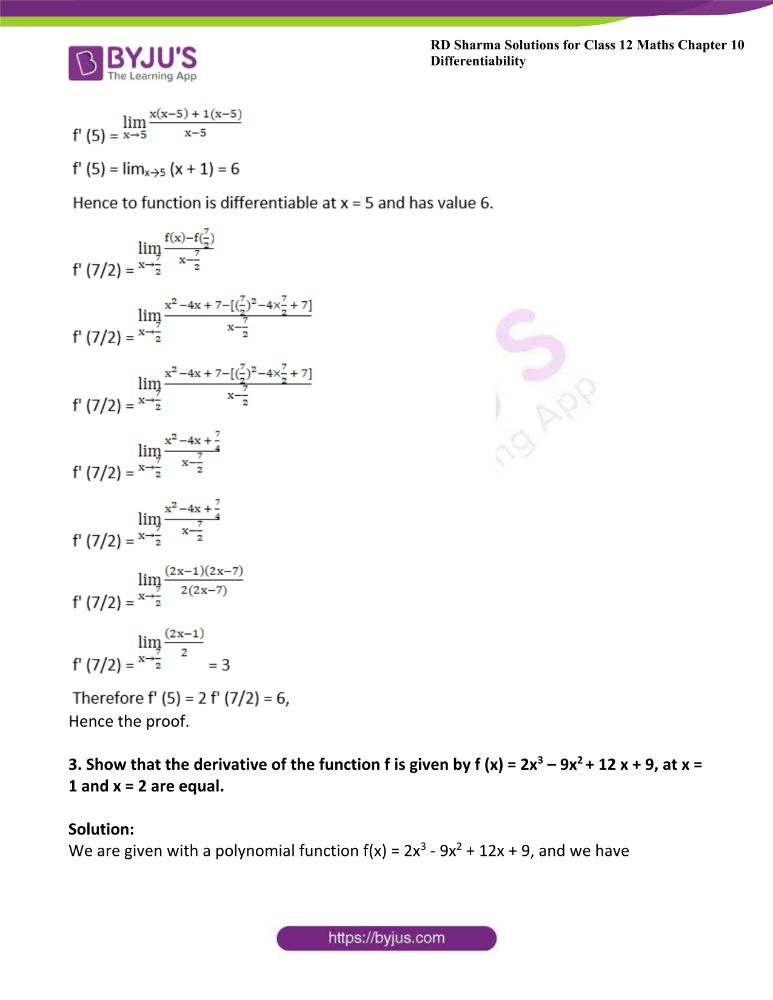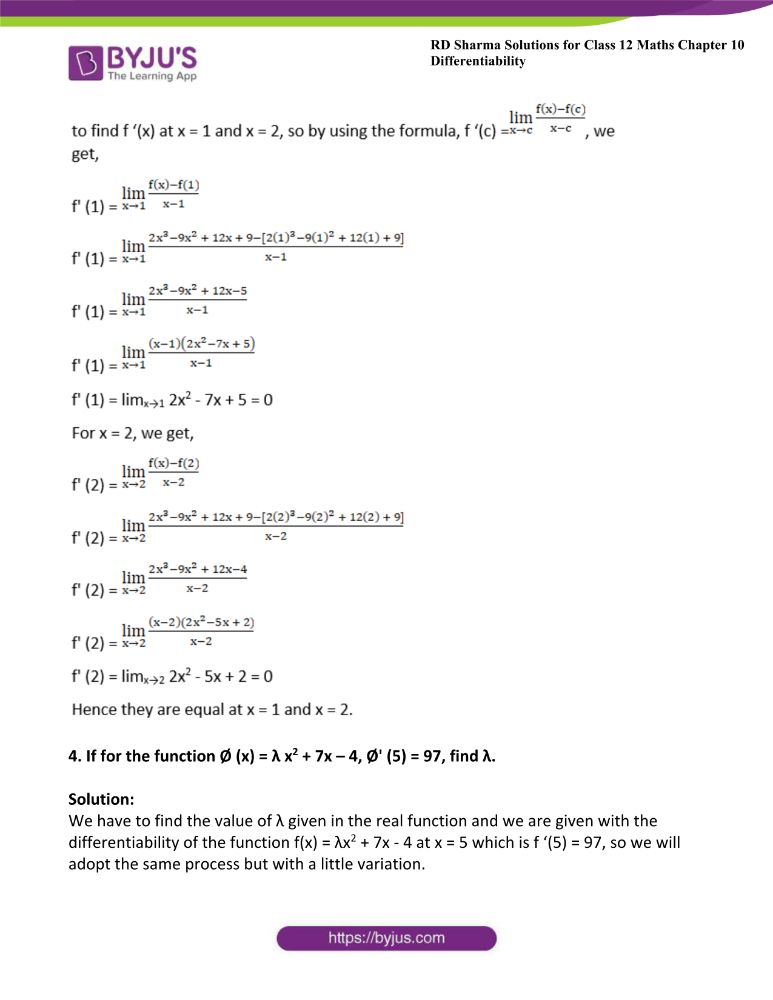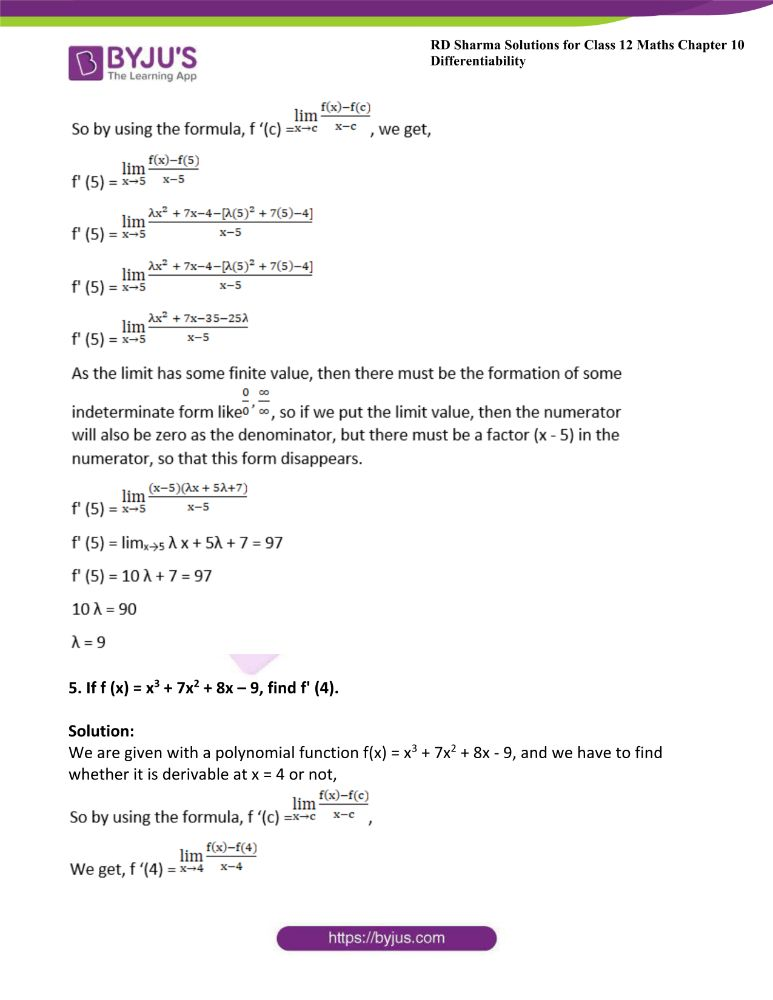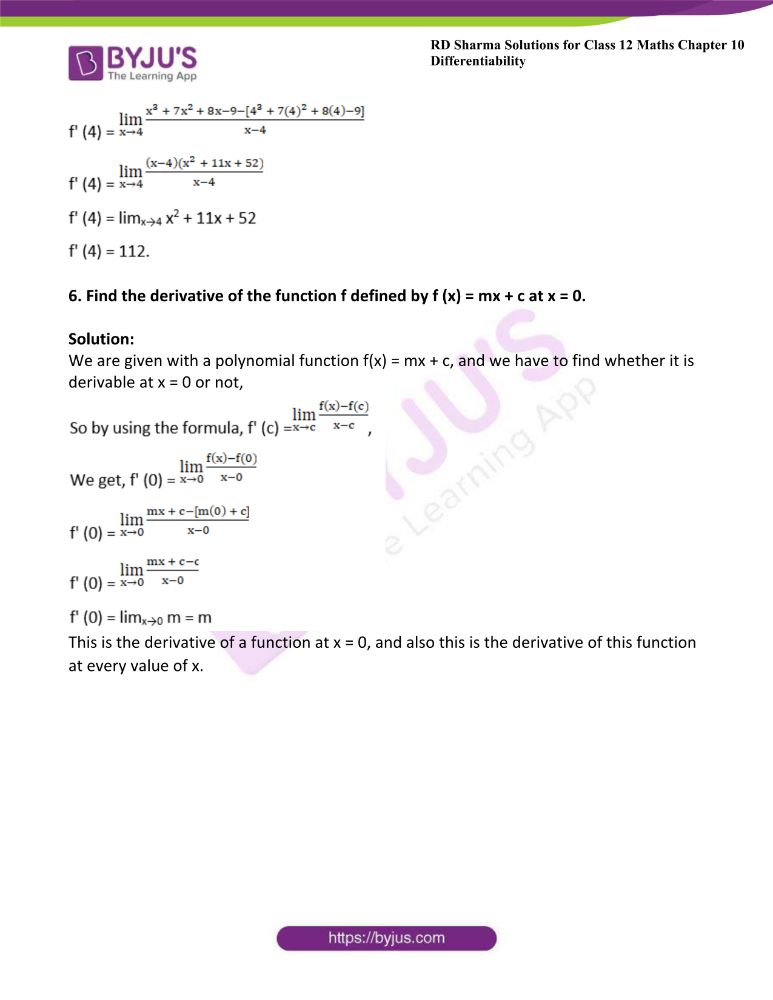### Access answers to Maths RD Sharma Solutions For Class 12 Chapter 10 – Differentiability

Exercise 10.1 Page No: 10.10

1. Show that f (x) = |x – 3| is continuous but not differentiable at x = 3.

Solution: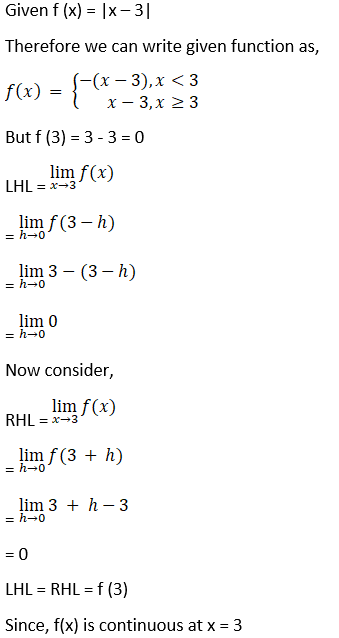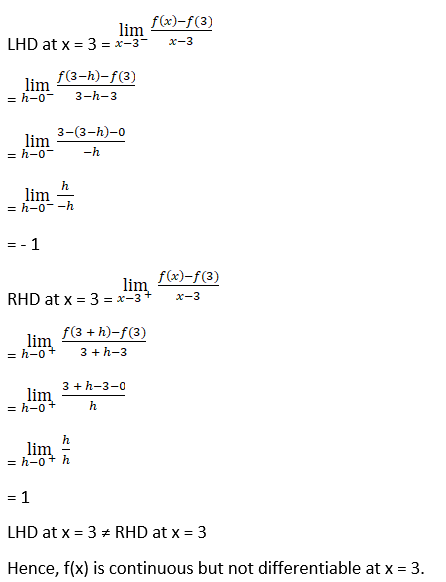2. Show that f (x) = x 1/3 is not differentiable at x = 0.

Solution: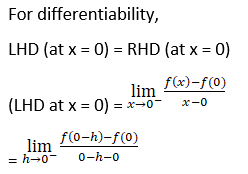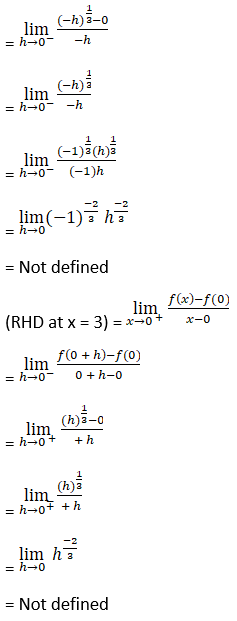Since, LHD and RHD does not exist at x = 0

Hence, f(x) is not differentiable at x = 0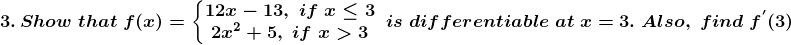Solution:

Now we have to check differentiability of given function at x = 3

That is LHD (at x = 3) = RHD (at x = 3)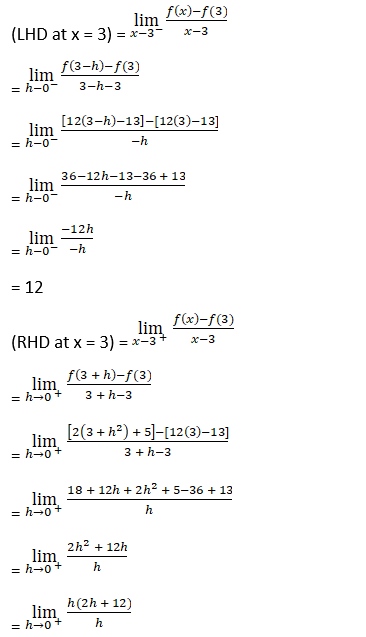= 12

Since, (LHD at x = 3) = (RHD at x = 3)

Hence, f(x) is differentiable at x = 3.

4. Show that the function f is defined as follows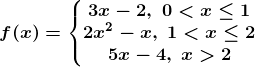Is continuous at x = 2, but not differentiable thereat.

Solution: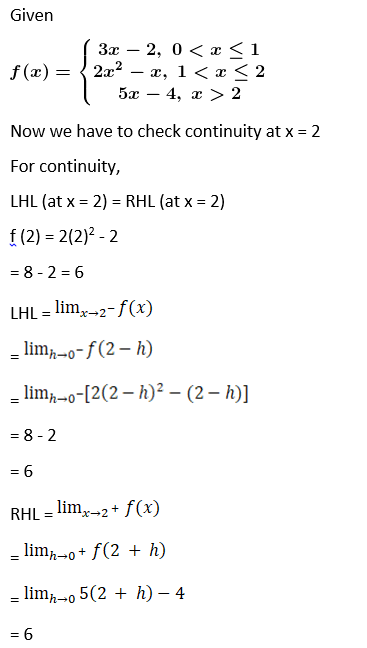Since, LHL = RHL = f (2)

Hence, F(x) is continuous at x = 2

Now we have to differentiability at x = 2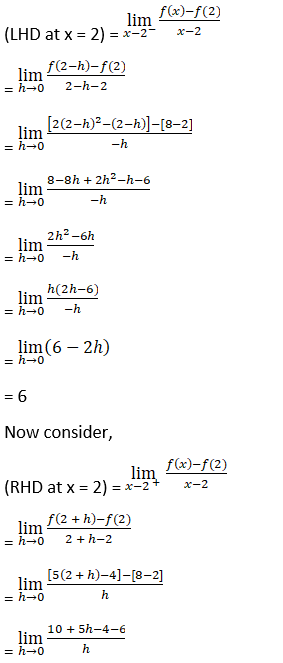= 5

Since, (RHD at x = 2) ≠ (LHD at x = 2)

Hence, f (2) is not differentiable at x = 2.

5. Discuss the continuity and differentiability of the function f (x) = |x| + |x -1| in the interval of (-1, 2).

Solution: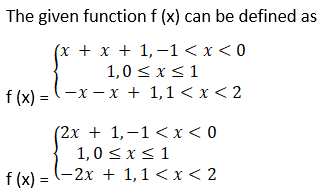We know that a polynomial and a constant function is continuous and differentiable everywhere. So, f(x) is continuous and differentiable for x ∈

(-1, 0) and x ∈ (0, 1) and (1, 2).

We need to check continuity and differentiability at x = 0 and x = 1.

Continuity at x = 0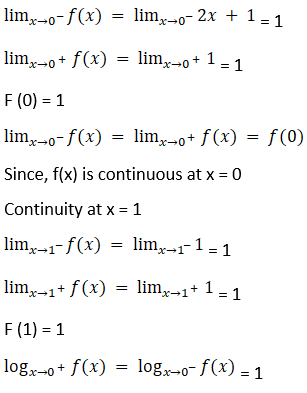Since, f(x) is continuous at x = 1

Now we have to check differentiability at x = 0

For differentiability, LHD (at x = 0) = RHD (at x = 0)

Differentiability at x = 0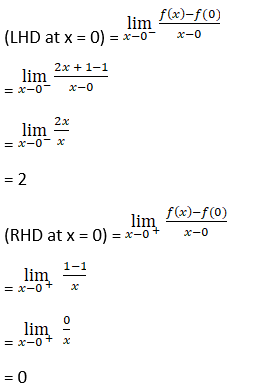Since, (LHD at x = 0) ≠ (RHD at x = 0)

So, f(x) is differentiable at x = 0.

Now we have to check differentiability at x = 1

For differentiability, LHD (at x = 1) = RHD (at x = 1)

Differentiability at x = 1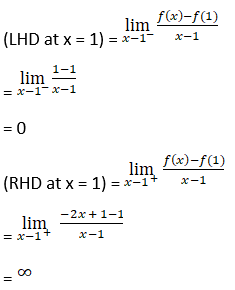Since, f(x) is not differentiable at x = 1.

So, f(x) is continuous on (- 1, 2) but not differentiable at x = 0, 1

Exercise 10.2 Page No: 10.16

1. If f is defined by f (x) = x2, find f’ (2).

Solution: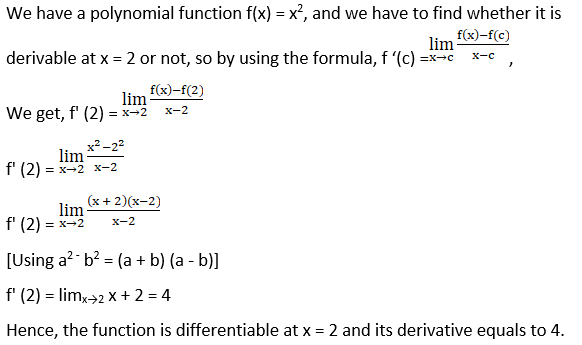2. If f is defined by f (x) = x2 – 4x + 7, show that f’ (5) = 2 f’ (7/2)

Solution: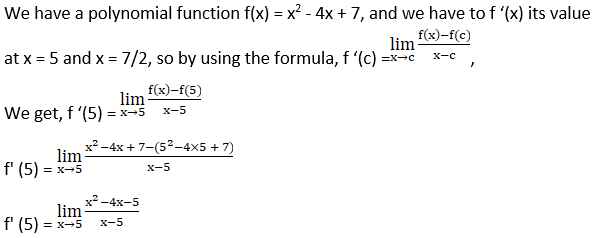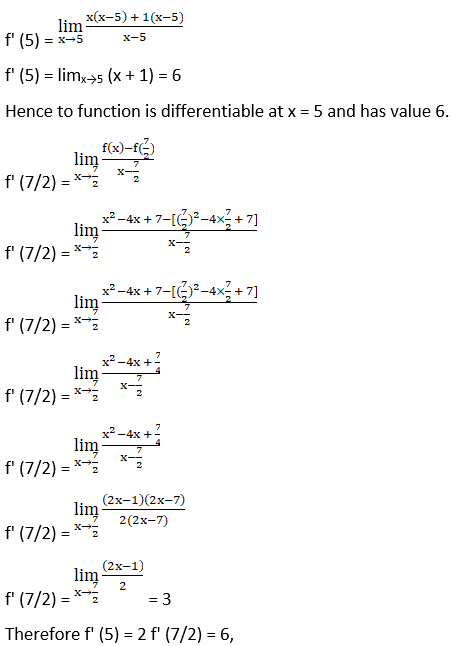Hence the proof.

3. Show that the derivative of the function f is given by f (x) = 2x3 – 9x2 + 12 x + 9, at x = 1 and x = 2 are equal.

Solution:

We are given with a polynomial function f(x) = 2x3 – 9x2 + 12x + 9, and we have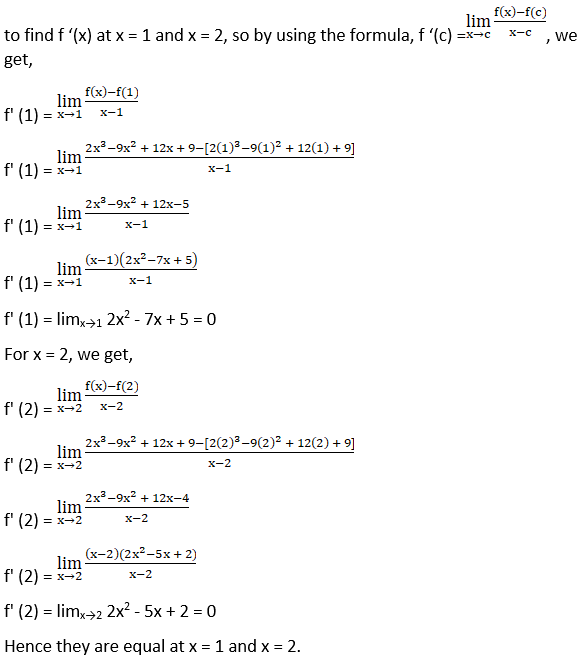4. If for the function Ø (x) = λ x2 + 7x – 4, Ø’ (5) = 97, find λ.

Solution:

We have to find the value of λ given in the real function and we are given with the differentiability of the function f(x) = λx2 + 7x – 4 at x = 5 which is f ‘(5) = 97, so we will adopt the same process but with a little variation.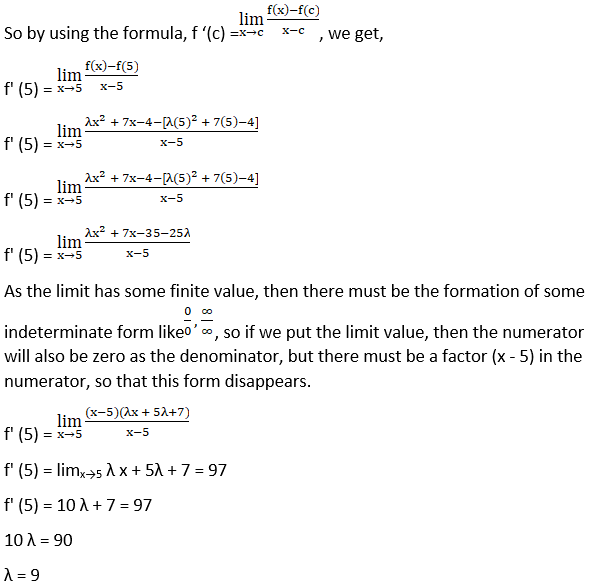5. If f (x) = x3 + 7x2 + 8x – 9, find f’ (4).

Solution:

We are given with a polynomial function f(x) = x3 + 7x2 + 8x – 9, and we have to find whether it is derivable at x = 4 or not,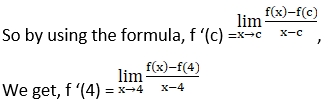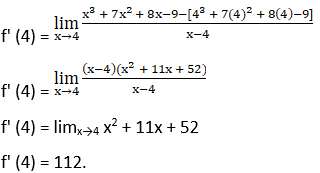6. Find the derivative of the function f defined by f (x) = mx + c at x = 0.

Solution:

We are given with a polynomial function f(x) = mx + c, and we have to find whether it is derivable at x = 0 or not,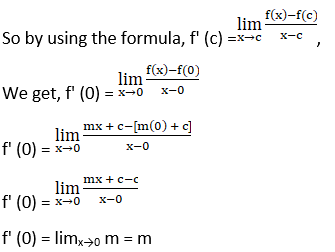This is the derivative of a function at x = 0, and also this is the derivative of this function at every value of x.

### Also, access RD Sharma Solutions for Class 12 Maths Chapter 10 Differentiability

Exercise 10.1 Solutions

## Frequently Asked Questions on RD Sharma Solutions for Class 12 Maths Chapter 10

### Is RD Sharma Solutions for Class 12 Maths Chapter 10 is the best reference guide for exam preparation?

Yes, RD Sharma Solutions for Class 12 Maths Chapter 10 is the best reference material for CBSE board exam preparation. Subject experts have framed and solved the questions from every section. Studying from these books will ensure that they can easily excel in their final exams. The books are completely based on the exam-oriented approach to help students score well in their board examinations. After completing the textbook questions, students can move on to the previous year question papers and sample papers for a better idea of the exam pattern.

### Where can I get the correct Solutions for RD Sharma Class 12 Maths Chapter 10?

Students and teachers can access BYJU’S official website for RD Sharma Solutions Class 12 Maths Chapter 10 which provides a wide range of study materials like textbooks, reference books, etc. The solutions are prepared with utmost care to help students understand the concepts easily and score well in their board exams.

### How to achieve good marks by referring to RD Sharma Class 12 Maths Chapter 10?

By referring to RD Sharma Solutions for Class 12 Maths Chapter 10 available at BYJU’S website, students can achieve high marks in the main exams. Students are required to go through RD Sharma Solutions thoroughly before the final exams to score well and intensify their question-solving abilities. Practice is an essential task to learn and score well in Maths.

### List out the concepts discussed in RD Sharma Solutions for Class 12 Maths Chapter 10

The concepts discussed in Chapter 10 of RD Sharma Solutions for Class 12 Maths are:
• Differentiability at a point
• Definition and meaning of differentiability at a point
• Differentiability in a set
• Some useful results on differentiability

### Why RD Sharma Solutions for Class 12 Maths Chapter 10 is beneficial for students to score good marks in exams?

RD Sharma Solutions for Class 12 Maths Chapter 10 is prepared by BYJU’S experts in accordance with understanding abilities of students. The solution PDF can be downloaded from BYJU’S based on the students needs. All the important concepts are explained in a simple and understandable language to help students ace the exam fearlessly. The precise solutions to all the questions present in the textbook help students to cross check their answers and analyze their area of weakness.